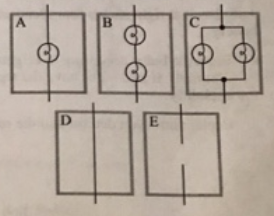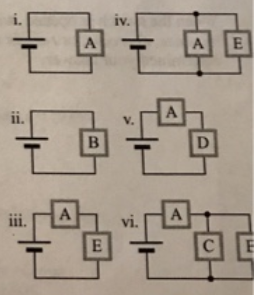# Problem: Consider the five networks shown at right.a. Rank the networks according to equivalent resistance, from largest to smallest. (Hint: Imagine placing each network in series with an indicator bulb and a battery.)b. How does adding a single bulb to a circuit in series with another bulb or network affect the resistance of the circuit?c. How does adding a single bulb to a circuit in parallel with another bulb or network affect the resistance of the circuit?d. The networks A-E are connected, in turn, to identical batteries as shown.Use the model we have developed to:• rank the circuits according to equivalent resistance, from largest to smallest. Explain.• rank the circuits according to the current through the battery, from largest to smallest. Explain.

###### FREE Expert Solution

Equivalent resistance for resistors in parallel:

$\overline{)\frac{\mathbf{1}}{{\mathbf{R}}_{\mathbf{eq}}}{\mathbf{=}}\frac{\mathbf{1}}{{\mathbf{R}}_{\mathbf{1}}}{\mathbf{+}}\frac{\mathbf{1}}{{\mathbf{R}}_{\mathbf{2}}}{\mathbf{+}}{\mathbf{.}}{\mathbf{.}}{\mathbf{.}}{\mathbf{+}}\frac{\mathbf{1}}{{\mathbf{R}}_{\mathbf{n}}}}$

or for 2 resistors:

$\overline{){{\mathbf{R}}}_{{\mathbf{eq}}}{\mathbf{=}}\frac{{\mathbf{R}}_{\mathbf{1}}{\mathbf{R}}_{\mathbf{2}}}{{\mathbf{R}}_{\mathbf{1}}\mathbf{+}{\mathbf{R}}_{\mathbf{2}}}}$

Equivalent resistance for resistors in series:

$\overline{){{\mathbf{R}}}_{{\mathbf{eq}}}{\mathbf{=}}{{\mathbf{R}}}_{{\mathbf{1}}}{\mathbf{+}}{{\mathbf{R}}}_{{\mathbf{2}}}{\mathbf{+}}{\mathbf{.}}{\mathbf{.}}{\mathbf{.}}{\mathbf{+}}{{\mathbf{R}}}_{{\mathbf{n}}}}$

a.

We'll assume that all the bulbs have equal resistance, R.

A is a single resistor. Resistance = R

B is two resistors in series.

Req = R1 + R2 = R + R = 2R

C is two resistors in parallel.

Req = R1R2/(R1 + R2) = R•R/(R + R) = R2/2R = R/2

D is a short circuit with zero resistance.

88% (98 ratings)###### Problem Details

Consider the five networks shown at right.a. Rank the networks according to equivalent resistance, from largest to smallest. (Hint: Imagine placing each network in series with an indicator bulb and a battery.)

b. How does adding a single bulb to a circuit in series with another bulb or network affect the resistance of the circuit?

c. How does adding a single bulb to a circuit in parallel with another bulb or network affect the resistance of the circuit?

d. The networks A-E are connected, in turn, to identical batteries as shown.Use the model we have developed to:

• rank the circuits according to equivalent resistance, from largest to smallest. Explain.

• rank the circuits according to the current through the battery, from largest to smallest. Explain.